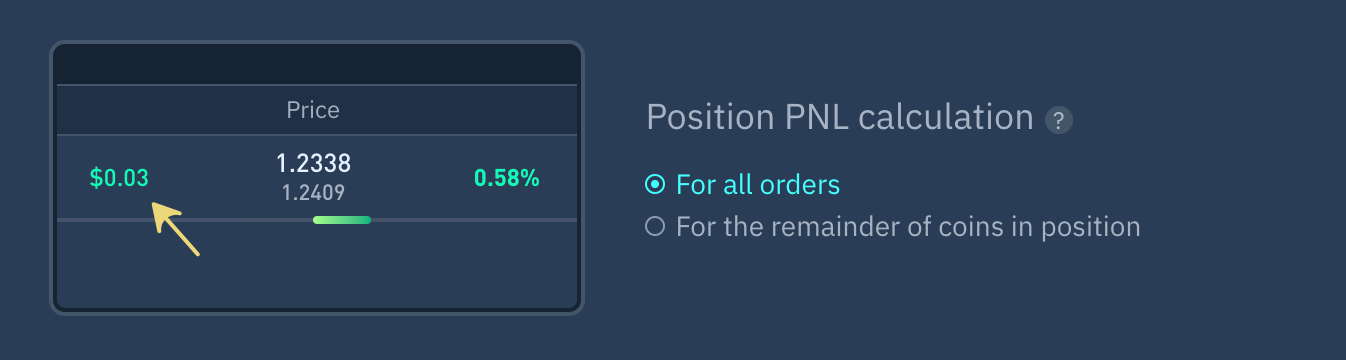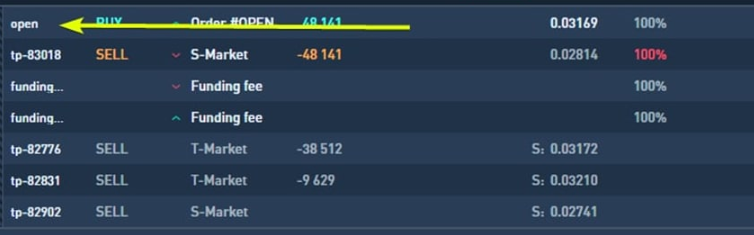# Position PNL Calculation

Last updated 16/01/2023
Profit calculation on all orders or by remainder of coins in positionThe process of calculating your PNL varies depending on the type of position you are in. Below are the formulas for each type.

## For all orders

PnL includes ALL realized profit/loss from partial closing of a position + profit/loss that would be approximately realized if the position were closed at the current price.
PNL = ( Partial close volume + Current price x Open position volume) x Direction - Commission + Funding
Direction = 1 if it is LONG Direction = -1 if it is SHORT
Example if exchange commission is 0.1%:
1. 1.
2. 2.
sells 0.8 BTC at price of 25 000 USDT (partial profit will be 4 000 excluding commissions)
PnL total in position at current exchange rate of 22 000 USDT will be calculated as follows: PnL = (25 000 x 0.8 + 22 000 x 0.2 - 20 000 x 1 ) x 1 - ( 25 000 x 0.8 + 22 000 x 0.2 + 20 000 x 1 ) x 0.001 = 4355.6 USDT There is no funding rate in this example, but if there were, it would increase or decrease the profit, depending on the sign of the funding amount

## For the remainder of coins in position

PnL is only calculated for the remaining coins in a position if the position is closed at the current price.PNL = ( Current Price - Position Price) x Quantity in Position) - Commission + Funding
Direction = 1 if it is LONG Direction = -1 if this is SHORT
Example with 0.1% commission
1. 1.
2. 2.
sells 0.8 BTC at price of 25 000 USDT (partial profit will be 4 000 excluding commissions)
3. 3.
Position price is equal to entry price (if the formula for position price calculation without partial closing is included)
PnL for a balance of 0.2 BTC at the current exchange rate of 22,000 USDT will be calculated as follows: PnL = (22 000 - 20 000) x 0.2 - 22 000 x 0.2 x 0.001 x 2 = 391.2 USDT There is no funding rate in this example, but if there were, it would increase or decrease the profit, depending on the sign of the funding amount